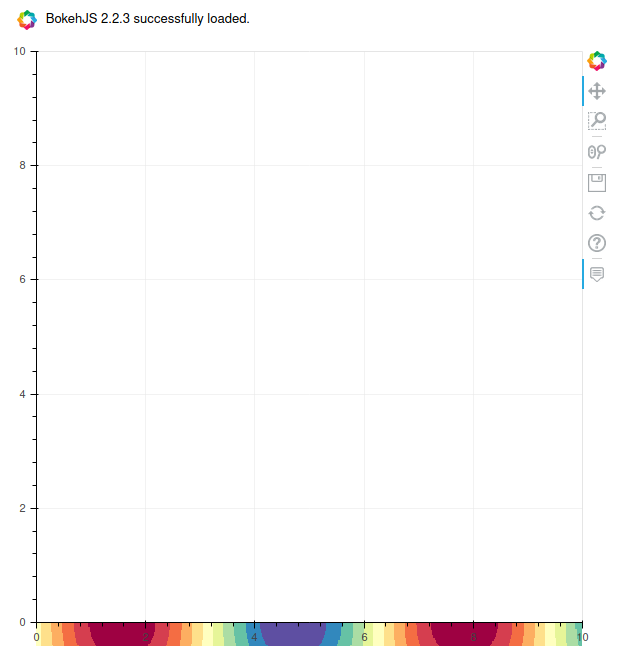/ bokeh Public

# [BUG] SVG backend mirrors image in plot#10725

Closed
opened this issue Nov 24, 2020 · 0 comments · Fixed by #10726
Closed

# [BUG] SVG backend mirrors image in plot #10725

opened this issue Nov 24, 2020 · 0 comments · Fixed by #10726
Labels
Milestone

### juhasch commented Nov 24, 2020

I want to export a Bokeh plot as SVG. Following https://docs.bokeh.org/en/latest/docs/user_guide/export.html, I add
`plot.output_backend = "svg"`. This causes the image in the plot to be mirrored and shifted outside of the bounding box.

• System: Linux Mint 20 Cinnamon, Firefox browser
• Anaconda installation with:
• bokeh 2.2.3 py_0 bokeh
• python 3.8.5 h1103e12_9_cpython conda-forge

This also happens with Windows + Anaconda + Edge browser

#### Example

Taken from the Bokeh gallery:

``````import numpy as np

from bokeh.plotting import figure, show
from bokeh.io import output_notebook
output_notebook()

N = 500
x = np.linspace(0, 10, N)
y = np.linspace(0, 10, N)
xx, yy = np.meshgrid(x, y)
d = np.sin(xx)*np.cos(yy)

p = figure(tooltips=[("x", "\$x"), ("y", "\$y"), ("value", "@image")])

# must give a vector of image data for image parameter
p.image(image=[d], x=0, y=0, dw=10, dh=10, palette="Spectral11", level="image")
p.grid.grid_line_width = 0.5

p.output_backend = "svg"  # SVG output
show(p)

``````